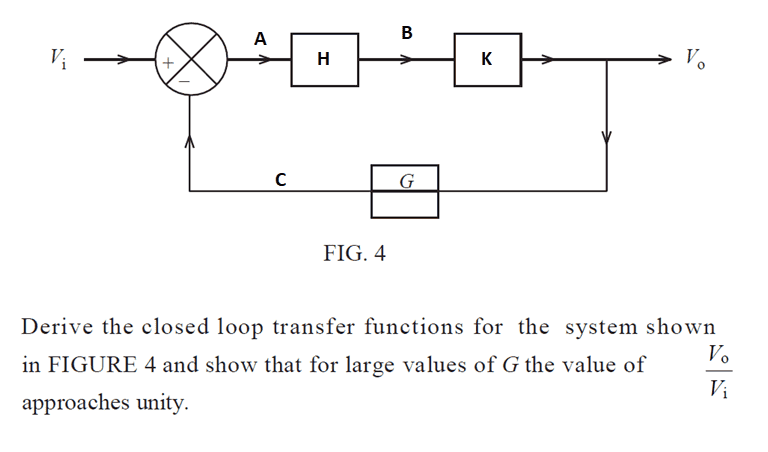# Closed Loop Transfer function

Jason-Li
Homework Statement:
Derive the closed loop transfer function for the system shown in FIGURE 4 and show that for large values of G the value of Vo/Vi approaches unity.
Relevant Equations:
Transfer Functions usedHi all Physics Forum User, I am again coming to you to ask for help.

For the above question I can't make sense of how a larger G will cause Vo/Vi to approach 1. For this I'll call Vo=V and Vi=I

As can be seen from the control loop

B = A*H and V = B*K hence V = A*H*K

Also A = I - C and C=Vo*G therefore A = I - V*G

Then substituting in V =(I-V*G)*HK
Hence Vo/Vi = (H*K) / (1+H*K*G)

Increasing G in this would have an opposite effect on making Vo/Vi approach unity.

Can anyone shed any light on this?

#### Attachments

•Delta2

Homework Helper
Gold Member
I'm not sure if this means anything, but I thought I would throw it out there: The labels in the figure are, well, unconventional.

There is a convention in many control systems textbooks that "G" is what's called the "plant," the operator taken in the feed-forward path.

And likewise, "H" is conventionally chosen to represent the operator in the feedback path.

For whatever reason, the figure in this problem has that sort of reversed. There's also a horizontal line that goes through the block in the feedback path, and I'm not sure if that's just an artistic choice or what.

Of course, that's purely a convention and it shouldn't change the underlying mathematics if that convention is not used.

I guess my question is are you sure the figure in Figure 4 is the same block diagram for the problem statement? Something about it seems a bit unconventional. Maybe there is some sort of mismatch between the problem statmement and the figure.

Jason-Li
I'm not sure if this means anything, but I thought I would throw it out there: The labels in the figure are, well, unconventional.

There is a convention in many control systems textbooks that "G" is what's called the "plant," the operator taken in the feed-forward path.

And likewise, "H" is conventionally chosen to represent the operator in the feedback path.

For whatever reason, the figure in this problem has that sort of reversed. There's also a horizontal line that goes through the block in the feedback path, and I'm not sure if that's just an artistic choice or what.

Of course, that's purely a convention and it shouldn't change the underlying mathematics if that convention is not used.

I guess my question is are you sure the figure in Figure 4 is the same block diagram for the problem statement? Something about it seems a bit unconventional. Maybe there is some sort of mismatch between the problem statmement and the figure.

Hi collinsmark,

Yeah I've seen on other websites explanations of stuff like this and the lettering does seem a bit off!

But anyway yes, the figure is definitely the one corresponding to the question - that's why I'm so confused - the question doesn't seem to make sense!

If the question were to be asking about large values of H or K I would understand that but large values of G has a counter effect than what it's asking!

Homework Helper
Gold Member
Hi collinsmark,

Yeah I've seen on other websites explanations of stuff like this and the lettering does seem a bit off!

But anyway yes, the figure is definitely the one corresponding to the question - that's why I'm so confused - the question doesn't seem to make sense!

If the question were to be asking about large values of H or K I would understand that but large values of G has a counter effect than what it's asking!
Your analysis looks good to me, given how the diagram was labeled. My only guess is that the diagram was mislabeled somehow or another.

•Jason-Li
Jason-Li
Your analysis looks good to me, given how the diagram was labeled. My only guess is that the diagram was mislabeled somehow or another.
]To confirm, it was labelled incorrectly. Thank you again!